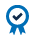##### Education Home

Fibonacci numbers have a foundation in ancient mathematics and have found their way in to trading indicators as well, included in most charting platforms.

The Fibonacci series of numbers is found by adding the two preceding numbers to find the next number. The series begins: 0,1,1,2,3,5,8,13…

For traders, the  important feature to note is that the ratio between numbers is close to 1:1.61, the Golden Ratio. For example, 8 divided by 5 = 1.60.

If you divide any number in the series by the number two spaces to the right, you get a ratio of 38.2. This can also be obtained by subtracting .618 from 1.

The third common ratio for retracements is 50%, this is not a number from the Fibonacci series of numbers but is included in the analysis when analyzing financial markets.

These numbers, 61.8 and 38.2 ratios along with the 50%, form the basis of Fibonacci studies of a product.

## Fibonacci Analysis

Traders use Fibonacci analysis to predict how far a stock might retrace a given move. By looking at the prior highs and lows of the previous move, traders use Fibonacci retracements to determine how far a current retracement in the stock might last.

The ratios form the basis to determine the size of the retracement. 38.2% is considered shallow retracement, while a retracement to the 62.8% is considered deeper. A 50% retracement is considered medium.

These three zones serve as alerts for the trader to watch for possible areas of price resuming its previous trend.

## Charting Breakout Extensions

Fibonacci ratios can also be used by traders to chart the potential extension of breakouts. These ratios take a percentage of the prior move’s distance and add that distance to where the stock is trading now.

For example, if the stock is trading at \$300 and 61.8% of the previous move is \$6, then a trader might look at \$306 as a target price for the stock and think about exiting if the price reaches that level. Because, at this level, the Fibonacci extension theory suggests that price might retrace and move lower.

The common extensions that are used by traders are the same as the retracements ratios and are 1.61, 1.50 and 1.38. These levels can help traders determine potential zones for the current breakout to end and change direction, or a zone for price to have a small pull back then resume its trend.

While most traders use Fibonacci ratios to identify price moves, these patterns and ratios can also be applied to time. The theory is that large moves in the market are followed by subsequent large moves in the market around set time periods. The time periods following the initial large move are based on Fibonacci numbers. 1,2,3,5,8,13,21,34, 55, 89, 144, 233 where the reference is to the number of days since the initial move. Typically, the analysis might start at 13 or 21 days.

## Conclusion

Fibonacci analysis can be summarized as identifying previous patterns in price and watching for those patterns to repeat. The theory also follows stock price moves and assumes they move up and down following the golden ratio.

However, one should use Fibonacci ratios like any piece of technical analysis. It should be used with other indicators and used as evidence to form an opinion not in isolation. Traders should remember that support and resistance levels fall and can be influenced by external events that shock the market and move price outside of its previous patterns.

#### ACCREDITED COURSEDid you know that CME Institute classes can fulfill CFA and GARP continuing education requirements? Every CME Institute course can be self-reported in your CFA online CE tracker and select classes can be used for GARP credits. See which of our classes qualify for GARP credits here.